Chapter 11: Exercises

1. Prove that the angle between two vectors $\mathbf{x}$ and $\mathbf{y}$ is $\cos^{-1}\frac{\mathbf{x}^T\mathbf{y}}{\|\mathbf{x}\|\|\mathbf{y}\|}$.
2. Prove that the projection of vector $\mathbf{x}$ onto vector $\mathbf{y}$ is $\frac{\mathbf{x}^T\mathbf{y}}{\|\mathbf{y}\|}$.
1. What is the goal of PCA?
2. Please write down the objective function of PCA.
3. Please derive the first principal component of PCA.
4. Please derive the second principal component of PCA.
3. (**)Properties of symmetric square matrices: Prove the following properties of symmetric square matrices:
1. A square symmetric matrix have orthogonal eigenvectors corresponding to different eigenvalues.
4. (*)About PCA computation: If A is a 10000-by-400 real matrix. Explain how you can compute the 400 largest eigenvalues (and the corresponding eigenvectors) of AA' without computing AA' in advance.
5. (*)The shortest distance between a point and a line in 2D: Prove that the shortest distance between a point $(x_0, y_0)$ and a line $ax+by+c=0$ in a 2D space is $\frac{|ax_0+by_0+c|}{\sqrt{a^2+b^2}}$.
6. (*)PCA for line fitting of total least-squares in 2D: Given a dataset of X-Y pairs $\{(x_i, y_i)|i=1, \dots, n\}$, the goal of total least-squares estimate is to find a line $ax+by+c=0$ such that the sum of shortest squared distances of all points to the line is minimized. The objective function can be expressed as $$J(a, b, c) = \sum_{i=1}^n \frac{\left(ax_i+by_i+c\right)^2}{a^2+b^2}$$ Prove that the line must cross the dataset's mean point $\left(\mu_x, \mu_y\right) = \left(\frac{\sum_{i=1}^n x_i}{n}, \frac{\sum_{i=1}^n y_i}{n} \right)$.
Hint: $\frac{\partial J}{\partial c}=0$.
7. (*)The shortest distance between a point and a plane in 3D: Prove that the shortest distance between a point $(x_0, y_0, z_0)$ and a plane $ax+by+cz+d=0$ in a 3D space is $\frac{|ax_0+by_0+cz_0+d|}{\sqrt{a^2+b^2+c^2}}$.
8. (*)PCA for plane fitting of total least-squares in 3D: Given a dataset of X-Y-Z points $\{(x_i, y_i, z_i)|i=1, \dots, n\}$, the goal of total least-squares estimate is to find a plane $ax+by+cz+d=0$ such that the sum of shortest squared distances of all points to the plane is minimized. The objective function can be expressed as $$J(a, b, c, d) = \sum_{i=1}^n \frac{\left(ax_i+by_i+cz_i+d\right)^2}{a^2+b^2+c^2}$$ Prove that the plane must cross the dataset's mean point $\left(\mu_x, \mu_y, \mu_z\right) = \left(\frac{\sum_{i=1}^n x_i}{n}, \frac{\sum_{i=1}^n y_i}{n}, \frac{\sum_{i=1}^n z_i}{n} \right)$.
Hint: $\frac{\partial J}{\partial d}=0$.
9. (*)The shortest distance between a point and a line in 3D space: Prove that the square of the shortest distance between a point $(x_0, y_0, z_0)$ and a line $\frac{x-p}{a}=\frac{y-q}{b}=\frac{z-r}{c}$ in a 3D space is $(x_0-p)^2+(y_0-q)^2+(z_0-r)^2-\frac{\left[a(x_0-p)+b(y_0-q)+c(z_0-r)\right]^2}{a^2+b^2+c^2}$.
10. (*)PCA for line fit of total least-squares in 3D: Given a dataset of X-Y-Z points $\{(x_i, y_i, z_i)|i=1, \dots, n\}$, the goal of total least-squares estimate for a line is to find a line $\frac{x-p}{a}=\frac{y-q}{b}=\frac{z-r}{c}$ such that the sum of shortest squared distances of all points to the line is minimized. The objective function can be expressed as $$J(p, q, r, a, b, c) = \sum_{i=1}^n \left\{ (x_i-p)^2+(y_i-q)^2+(z_i-r)^2 - \frac{\left[ a(x_i-p)+b(y_i-q)+c(z_i-r)\right]^2}{a^2+b^2+c^2} \right\}$$ (See the previous exercise.) Prove that the line must cross the dataset's mean point $\left(\mu_x, \mu_y, \mu_z\right) = \left(\frac{\sum_{i=1}^n x_i}{n}, \frac{\sum_{i=1}^n y_i}{n}, \frac{\sum_{i=1}^n z_i}{n} \right)$.
Hint: $\frac{\partial J}{\partial p}=\frac{\partial J}{\partial q}=\frac{\partial J}{\partial r}=0 \Rightarrow \frac{\mu_x-p}{a}=\frac{\mu_y-q}{b}=\frac{\mu_z-r}{c}$
11. (*)Difference between LSE and TLS: What is the key difference between LSE (least-squares estimate) and TLS (total least-squares)?
12. (*)Strength and weakness of TLS: Please give the strength and weakness of TLS (total least-squares) when compared with the common LSE (least-squares estimate).
13. (*)Function for line fitting via TLS: Write a function that performs TLS for line fitting, with the following usage:
coef=lineFitViaTls(data)
where
• data: A 2-row data matrix with row 1 as the x and row 2 as the y.
• coef: A 3-element column vector of the coefficients [a, b, c] of the fitting line $ax+by+c=0$, satisfying $a^2+b^2=1$. (Note that in order to make the identified coefficients unique, the first non-zero coefficient must be positive.)
(Note that you need to write your own PCA code since it is straightforward. You are not allowed to use the "pca" command from anywhere else.)

You can download the p-file lineFitViaTls.p to test your function. Here is a test case to show the difference between TLS and LS:

Example 1: lineFitViaTls01.mx=[1.44 2.27 4.12 3.04 5.13 7.01 7.01 10.15 8.30 9.88]; y=[8.20 11.12 14.31 17.78 17.07 21.95 25.11 30.19 30.95 36.05]; data=[x; y]; theta=lineFitViaTls(data); % TLS fitting x2=linspace(min(x), max(x)); x2=linspace(min(x), max(x)); y2=(-theta(1)*x2-theta(3))/theta(2); % y=(-ax-c)/b plot(x, y, 'ro', x2, y2, 'b-'); axis image % LS fitting A=[x', ones(length(x), 1)]; theta2=A\y'; y3=theta2(1)*x2+theta2(2); % y=a*x+b line(x2, y3, 'color', 'm'); title('Line fit via TLS & LS'); legend('Sample data', 'TLS', 'LS', 'location', 'northOutside');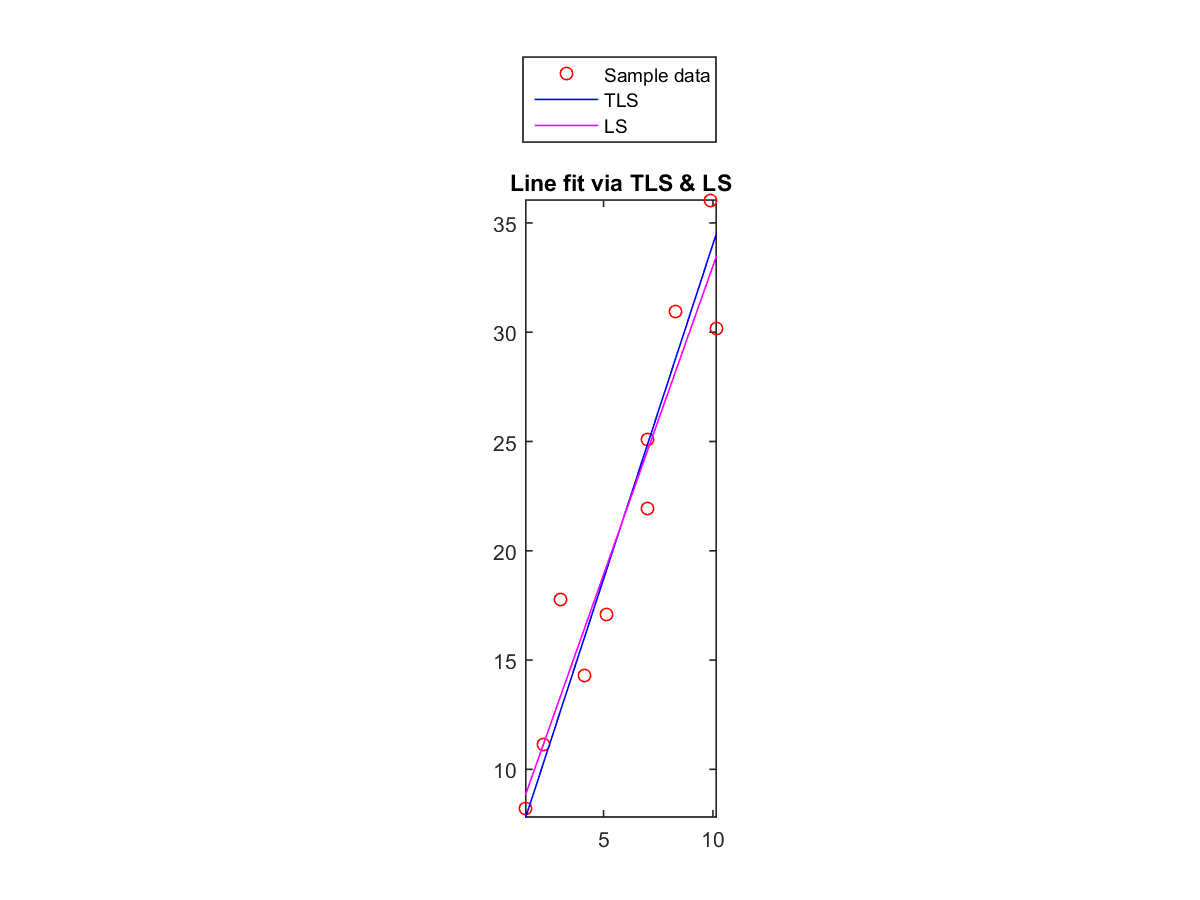14. (*)Function for plane fitting via TLS: Write a function that performs TLS for plane fitting, with the following usage:
coef=planeFitViaTls(data)
where
• data: A 3-row data matrix with row 1 as the x, row 2 as the y, and row 3 as the z.
• coef: A 4-element column vector of the coefficients [a, b, c, d] of the fitting plane $ax+by+cz+d=0$, satisfying $a^2+b^2+c^2=1$. (Note that in order to make the identified coefficients unique, the first non-zero coefficient must be positive.)
(Note that you need to write your own PCA code since it is straightforward. You are not allowed to use the "pca" command from anywhere else.)

Example 2: planeFitViaTls01.m%x=10*rand(1,100)-5; %y=10*rand(1,100)-5; %z=x+2*y+3+randn(size(x)); %data=round([x; y; z]); data=[2 -5 -4 0 -4 3 3 2 -4 2 0 5 1 3 0 -1 3 -4 -4 -3 -1 3 3 -4 -1 0 -1 2 1 -2 -1 -5 5 -3 -4 -1 -3 0 -2 5 4 -4 2 -2 -1 0 4 -1 5 -2 2 2 0 2 2 -3 -4 5 -3 -5 1 4 2 -3 -1 0 5 -3 4 1 -1 -3 -1 0 -4 1 -3 -1 1 -2 -2 1 -2 3 5 2 -2 1 -4 4 4 3 -2 1 -5 -1 -2 -3 -3 -1; -4 1 0 2 2 1 -5 -4 -2 0 2 -1 3 2 5 0 -2 -4 1 3 -1 -4 -2 -3 -2 -1 0 0 4 0 4 1 5 -3 2 -2 2 2 -4 -2 -3 2 3 -2 3 2 -5 1 -1 4 -5 0 -1 0 3 -2 3 0 -5 -3 2 0 -3 -2 1 -3 2 -3 4 -2 3 -3 -2 -4 1 2 0 -1 1 1 2 1 4 -3 2 -3 -4 1 0 0 2 3 -1 2 -1 3 3 -2 1 1; -3 2 -2 6 1 10 -2 -3 -3 5 7 6 11 10 15 2 1 -10 0 5 0 -2 2 -8 -2 4 4 2 9 2 9 1 17 -3 3 -3 4 7 -7 2 0 2 11 -3 9 7 -3 5 5 10 -5 5 3 4 9 -5 5 7 -10 -7 8 7 -3 -2 3 -3 14 -6 14 2 8 -7 -1 -6 1 9 0 1 8 4 6 7 8 -2 12 1 -6 6 -2 6 8 11 -3 8 -3 8 8 -6 1 5]; coef=planeFitViaTls(data); % Plot the result x2=linspace(min(data(1,:)), max(data(1,:))); y2=linspace(min(data(2,:)), max(data(2,:))); [xx2, yy2]=meshgrid(x2, y2); zz2=(-coef(1)*xx2-coef(2)*yy2-coef(4))/coef(3); mesh(xx2, yy2, zz2); axis tight; for i=1:size(data,2) line(data(1,i), data(2,i), data(3, i), 'marker', 'o'); end title('Plane fit via total least-squares');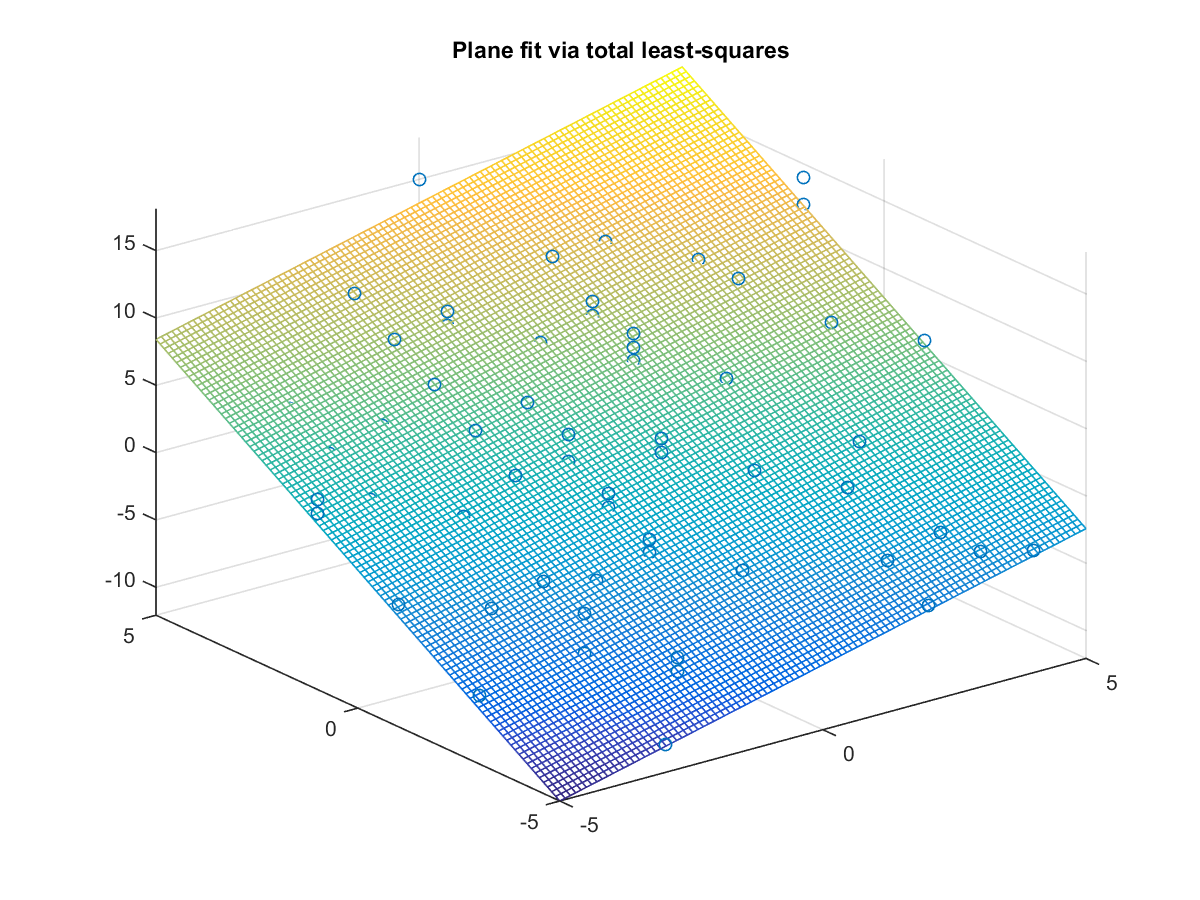15. (*)Function for hyperplane fitting via TLS: Write a function that performs TLS for hyperplane fitting, with the following usage:
coef=hyperplaneFitViaTls(data)
where
• data: A $d$-row data matrix with row 1 as the $x_1$, row 2 as the $x_2$, and so on.
• coef: A ($d+1$)-element column vector of the coefficients $[a_1, a_2, \dots a_d, a_{d+1}]$ of the fitting plane $a_1x_1+a_2x_2+\cdots+a_dx_d+a_{d+1}=0$, satisfying $\sum_{i=1}^{d}a_i^2=1$. (Note that in order to make the identified coefficients unique, the first non-zero coefficient must be positive.)
(Note that you need to write your own PCA code since it is straightforward. You are not allowed to use the "pca" command from anywhere else.)

Example 3: hyperplaneFitViaTls01.m%x=10*rand(1,100)-5; %y=10*rand(1,100)-5; %z=10*rand(1,100)-5; %u=x+2*y+3*z+4+randn(size(x)); %data=round([x; y; z; u]); data=[1 4 2 1 3 4 5 -5 4 1 5 0 0 3 -3 0 4 1 3 2 1 -3 2 -4 1 2 2 4 5 3 1 4 1 -5 -4 4 0 3 -3 1 1 -5 1 -1 -5 0 -3 -4 -3 -4 -3 -5 1 -2 0 2 0 0 -1 -4 0 4 4 -2 -3 1 1 -1 -3 4 -4 -4 -4 -3 1 1 -4 4 2 2 -4 4 4 5 4 3 0 -3 -1 -4 -5 4 -2 -2 -2 0 1 -5 3 1; 4 -2 -1 -4 -3 2 -2 4 -4 5 0 2 5 -2 -1 0 3 3 -4 -3 -1 -4 0 -2 -3 -3 4 2 0 4 -4 2 2 1 -3 1 -2 -4 -3 4 -4 -3 -4 -1 -5 4 -3 -4 -2 0 -4 5 -2 -2 -4 -2 -5 0 3 1 -4 -4 3 4 0 -4 3 -2 -2 2 -5 -5 2 1 0 2 2 3 -2 2 1 -1 -4 3 -2 1 2 -4 -4 0 0 4 3 2 -4 -4 -4 3 4 2; -4 2 -4 -4 1 -2 2 2 1 2 -3 2 5 4 -4 -1 -1 2 1 3 -1 -3 -4 3 -3 -1 1 -3 1 0 -3 3 -4 -2 -3 0 -4 -1 -4 -4 3 -2 1 5 -1 2 3 -1 2 -4 4 -3 -2 3 0 3 -1 -2 -5 2 -1 0 1 -4 -2 3 2 -4 -4 -4 -5 -1 2 2 0 -4 1 -4 -4 -4 -4 -3 -3 -2 -2 -3 -2 4 2 1 -3 -3 -4 4 2 1 -2 -3 1 5; -1 11 -7 -18 6 5 12 14 3 21 1 14 28 15 -13 0 9 17 4 9 -3 -17 -6 5 -11 -3 17 3 12 12 -14 20 -3 -5 -12 12 -13 -3 -16 1 6 -11 -2 16 -12 17 2 -9 1 -13 6 2 -5 7 -6 12 -8 -2 -6 9 -6 -3 17 -4 -4 5 18 -12 -14 2 -23 -12 11 11 7 -3 7 4 -10 -2 -9 -4 -9 9 -2 0 2 4 3 4 -10 8 -4 23 -1 -5 -6 -4 19 26]; coef=hyperplaneFitViaTls(data) coef = 0.2366 0.5200 0.7804 -0.2541 1.0762

Note that hyperplaneFitViaTls.m is more general and it should be used to replace lineFitViaTls.m and planeFitViaTls.m in the previous two exercises.

16. (*)比較LDA和PCA對於WINE辨識率的影響: 請寫一個程式 ldaPcaWineDim01.m，畫出兩條曲線，顯示在使用 LDA 和 PCA 時，辨識率（使用 KNNC 及 leave-one-out）對於資料維度的圖形。所畫出的圖形應該類似下圖：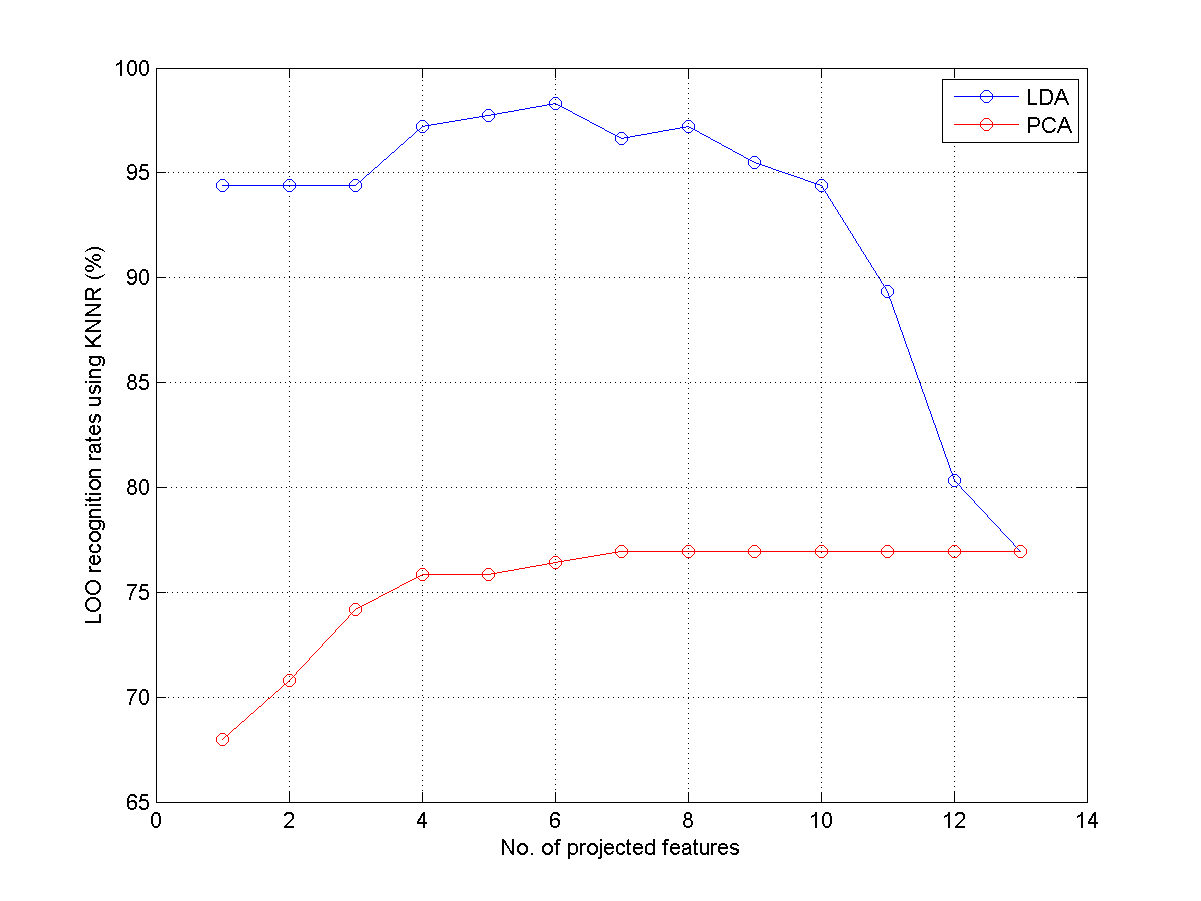17. (**)比較LDA和PCA對於WINE辨識率的影響: 請寫一個程式 ldaPcaWineDim02.m，畫出 4 條曲線，顯示在使用 LDA 和 PCA 時，以及是否使用資料正規化時，辨識率（使用 KNNC 及 leave-one-out）對於資料維度的圖形。所畫出的圖形應該類似下圖：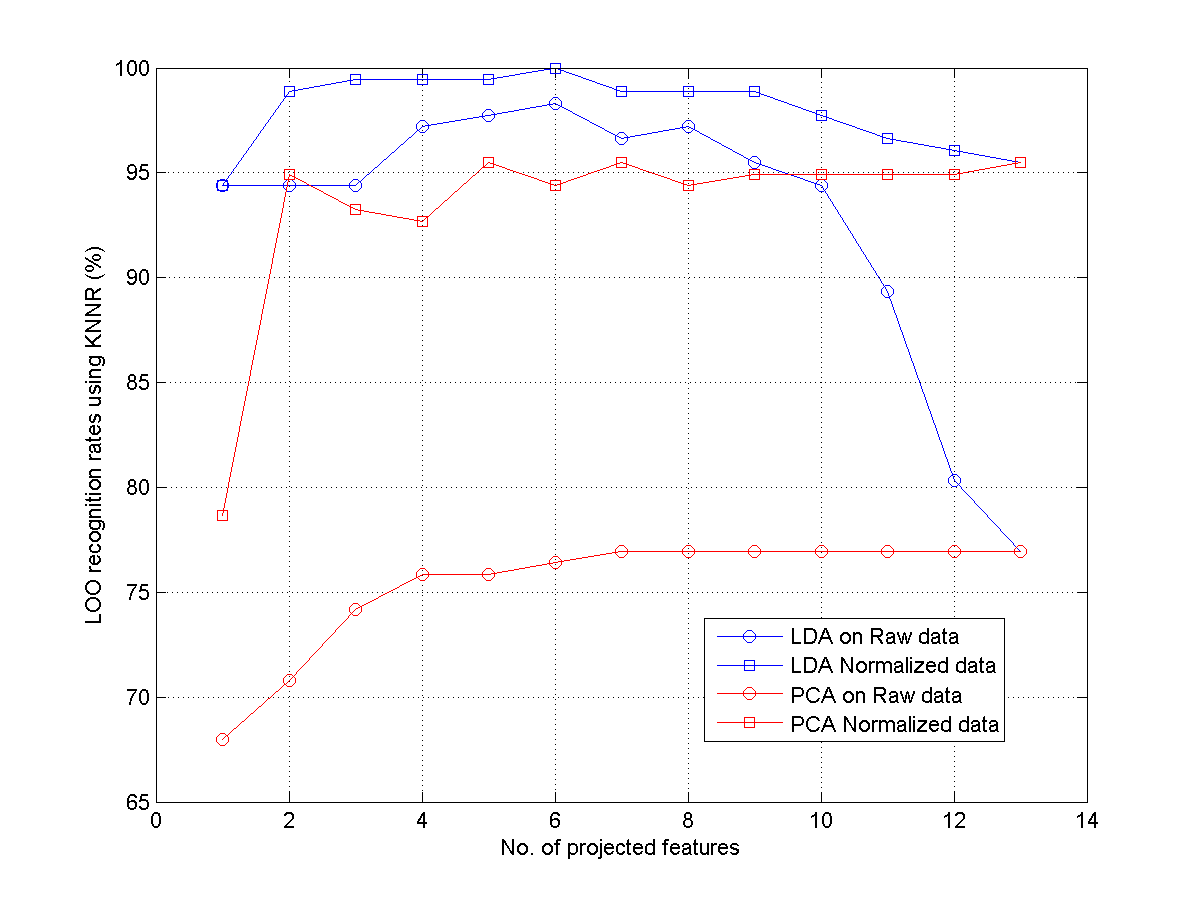18. (**)LDA用於母音資料的二維作圖: 請寫一個程式 ldaVowel2d01.m，使用 LDA 於此作業 「計算中文母音MFCC並進行分類」之母音資料，所用的語音特徵是12維的 MFCC，並畫出四個圖：
1. 使用原始 MFCC 資料，投影於 LDA 前兩維。
2. 使用原始 MFCC 資料，投影於 LDA 後兩維。
3. 使用正規化後的 MFCC 資料，投影於 LDA 前兩維。
4. 使用正規化後的 MFCC 資料，投影於 LDA 後兩維。
除了顯示圖形外，並顯示使用 KNNC 的 leave-one-out 辨識率。所畫出的圖形應該類似下圖：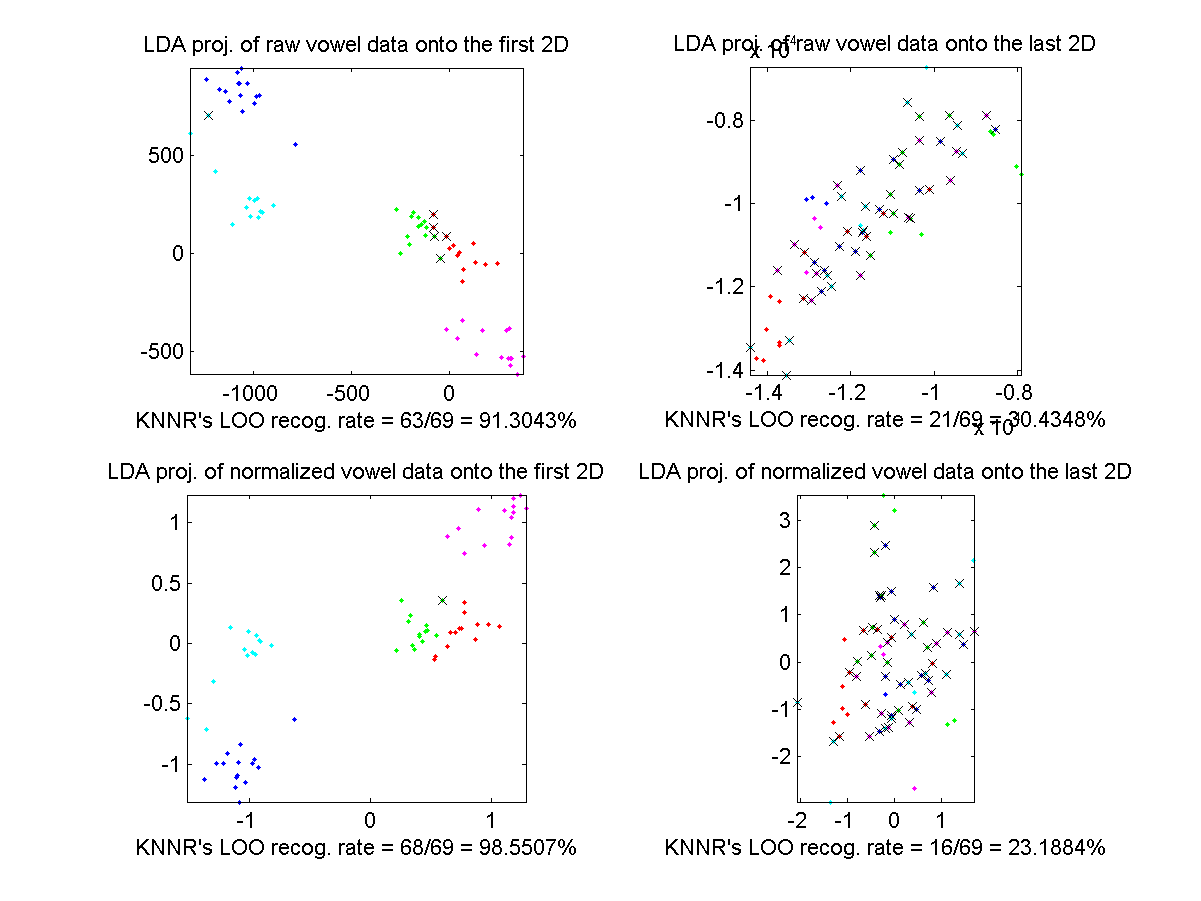19. (**)LDA用於母音資料並變化維度: 請寫一個程式 ldaVowelDim02.m，使用 LDA 於此作業 「計算中文母音MFCC並進行分類」之母音資料，所用的語音特徵是12維的 MFCC，並畫出辨識率（使用 KNNC 及 LOO）對 LDA 維度的曲線，應有兩條，代表使用原始及正規化之 MFCC 語音特徵。所畫出的圖形應該類似下圖：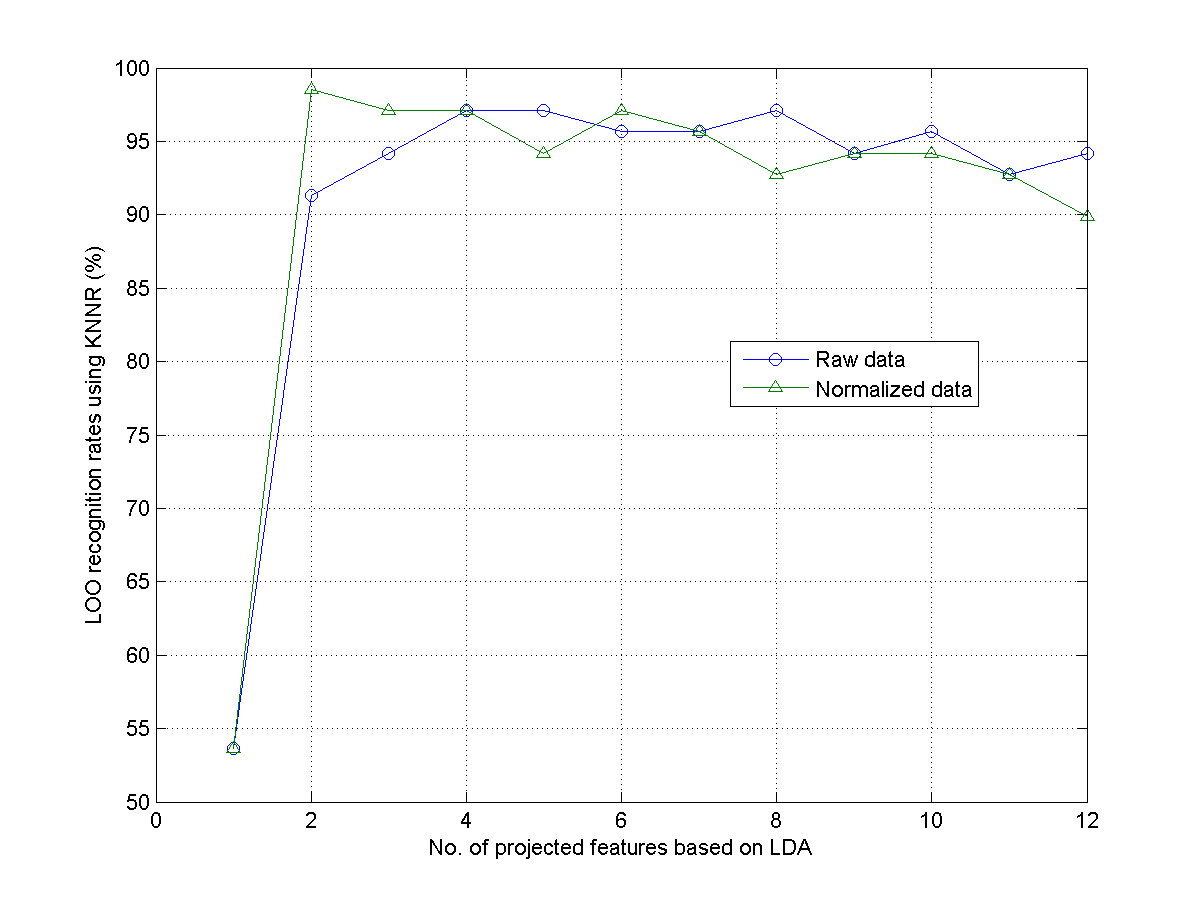20. (**)LDA用於母音資料並變化維度: 請寫一個程式 ldaVowelDim03.m，重複上題，但改用不同的語音特徵，看看是否能夠提高辨識率：
1. MFCC & log energy（共 13 維）。
2. MFCC & log energy, and their delta（共 26 維）。
3. MFCC & log energy, and their delta, and delta delta（共 39 維）。
21. (*)PCA for face recognition: Describe the basic steps of using PCA for face recognition. In particular, please explain what eigenfaces are and how they are used in the PCA-based approach.
22. (*)Display misclassified face images: In this example, we use PCA to obtain a recognition rate of 98.75%.
1. Display the misclassified 5 face images and their top-3 nearest neighbors.
2. Repeat part (a) using images after PCA+LDA projection.
23. (**)Feature selection after PCA for face recognition: PCA selects the principal components that explain the total variance most after projection. However, sometimes the variations are mostly due to lighting instead of differences among individuals. As a result, it becomes necessary to perform feature selection after PCA projection. This exercise explore such possibility by perform the following feature selection schemes after PCA projection.
1. Sequential feature selection.
2. Exhaustive feature selection. (Please limited the number of selected features based on your computer's capability.)
Please report the number of selected features and the corresponding accuracy.
24. (**)Class-specific PCA for face recognition: In this example, we use PCA to obtain a recognition rate of 98.75%. However, the projection is based on face images of all classes. Is it possible to have class-specific projections to have a better accuracy? Please design code to report your results and support your claims along this direction.

Data Clustering and Pattern Recognition (資料分群與樣式辨認)